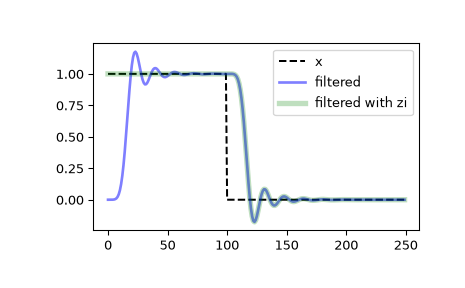# scipy.signal.sosfilt_zi¶

scipy.signal.sosfilt_zi(sos)[source]

Construct initial conditions for sosfilt for step response steady-state.

Compute an initial state zi for the `sosfilt` function that corresponds to the steady state of the step response.

A typical use of this function is to set the initial state so that the output of the filter starts at the same value as the first element of the signal to be filtered.

Parameters
sosarray_like

Array of second-order filter coefficients, must have shape `(n_sections, 6)`. See `sosfilt` for the SOS filter format specification.

Returns
zindarray

Initial conditions suitable for use with `sosfilt`, shape `(n_sections, 2)`.

Notes

New in version 0.16.0.

Examples

Filter a rectangular pulse that begins at time 0, with and without the use of the zi argument of `scipy.signal.sosfilt`.

```>>> from scipy import signal
>>> import matplotlib.pyplot as plt
```
```>>> sos = signal.butter(9, 0.125, output='sos')
>>> zi = signal.sosfilt_zi(sos)
>>> x = (np.arange(250) < 100).astype(int)
>>> f1 = signal.sosfilt(sos, x)
>>> f2, zo = signal.sosfilt(sos, x, zi=zi)
```
```>>> plt.plot(x, 'k--', label='x')
>>> plt.plot(f1, 'b', alpha=0.5, linewidth=2, label='filtered')
>>> plt.plot(f2, 'g', alpha=0.25, linewidth=4, label='filtered with zi')
>>> plt.legend(loc='best')
>>> plt.show()
```